# Algebra - math word problems

1. ChordIn a circle with radius r=60 cm is chord 4× longer than its distance from the center. What is the length of the chord?
2. RectanglesHow many rectangles with area 8713 cm2 whose sides is natural numbers are?
3. Guess numberWith what number should be divided number 9727 to be reduced by 70%?
4. Secret number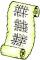Determine the secret number n, which reversed decrease by 16.4 if the number increase by 16.4.
5. AnglesThe triangle is one outer angle 158°54' and one internal angle 148°. Calculate the other internal angles of a triangle.
6. Rectangle SSPerimeter of a rectangle is 268 cm and its diagonal is 99.3 cm. Determine the dimensions of the rectangle.
7. TouristTourist walk at 4.1 km/h. Every 5 km have 20-minute break. How many kilometers passed, when started at 4:40 and finished at 10:30?
8. Heron backlawCalculate missing side in a triangle with sides 17 and 34 and area 275.
9. Seedcake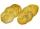Seedcake costs 44 cents. How many minimum seedcakes we must buy that we can pay in cash only whole euros?
10. CircleHow big is area of circle if its circumference is 80.6 cm?Quadratic equation ? has roots x1 = -26 and x2 = -86. Calculate the coefficients b and c.
12. NumberCalculate the integer number which, divided by 34 gives 10 and the rest 25.
13. Thrift woman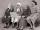Calculate how long grandmother will save to new shoes priced 108 euros if save 3 Eur monthly.
14. CapJesters hat is shaped by a rotating cone. Calculate how much paper is needed to the cap 54 cm high when the head circumference is 47 cm.
15. Compound interestCalculate time when deposit in the bank with interest 2.5% p.a. doubles.
16. Chestnuts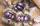Neil has 5-times chestnuts less than Adrian. Together they have 894 chestnuts. How many chestnuts has Neil and Adrian?
17. DemographicsThe population grew in the city in 10 years from 42000 to 54500. What is the average annual percentage increase of population?
18. Wood in the forest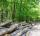The amount of wood in the forest was estimated at 6000 m3. How much wood will be in forest after 2 years if the annual growth of wood is 2.5% each year and logging 30 m3 each year?
19. Barrel of oilBarrel of oil weighs 283 kg. When it mold 26% oil, weighed 216 kg. What is the mass of the empty barrel?
20. Right triangleLegs of the right triangle are in the ratio a:b = 2:8. The hypotenuse has a length of 87 cm. Calculate the perimeter and area of the triangle.

Do you have an interesting mathematical word problem that you can't solve it? Submit math problem, and we can try to solve it.

We will send a solution to your e-mail address. Solved examples are also published here. Please enter the e-mail correctly and check whether you don't have a full mailbox.

Please do not submit problems from current active competitions such as Mathematical Olympiad, correspondence seminars etc...